Chapter 13 Temperature, Kinetic Theory, and the Gas Laws

# 93 13.1 Temperature

### Summary

• Define temperature.
• Convert temperatures between the Celsius, Fahrenheit, and Kelvin scales.
• Define thermal equilibrium.
• State the zeroth law of thermodynamics.

The concept of temperature has evolved from the common concepts of hot and cold. Human perception of what feels hot or cold is a relative one. For example, if you place one hand in hot water and the other in cold water, and then place both hands in tepid water, the tepid water will feel cool to the hand that was in hot water, and warm to the one that was in cold water. The scientific definition of temperature is less ambiguous than your senses of hot and cold. Temperature is operationally defined to be what we measure with a thermometer. (Many physical quantities are defined solely in terms of how they are measured. We shall see later how temperature is related to the kinetic energies of atoms and molecules, a more physical explanation.) Two accurate thermometers, one placed in hot water and the other in cold water, will show the hot water to have a higher temperature. If they are then placed in the tepid water, both will give identical readings (within measurement uncertainties). In this section, we discuss temperature, its measurement by thermometers, and its relationship to thermal equilibrium. Again, temperature is the quantity measured by a thermometer.

### MISCONCEPTION ALERT: HUMAN PERCEPTION VS. REALITY

On a cold winter morning, the wood on a porch feels warmer than the metal of your bike. The wood and bicycle are in thermal equilibrium with the outside air, and are thus the same temperature. They feel different because of the difference in the way that they conduct heat away from your skin. The metal conducts heat away from your body faster than the wood does (see more about conductivity in Chapter 14.5 Conduction). This is just one example demonstrating that the human sense of hot and cold is not determined by temperature alone.

Another factor that affects our perception of temperature is humidity. Most people feel much hotter on hot, humid days than on hot, dry days. This is because on humid days, sweat does not evaporate from the skin as efficiently as it does on dry days. It is the evaporation of sweat (or water from a sprinkler or pool) that cools us off.

Any physical property that depends on temperature, and whose response to temperature is reproducible, can be used as the basis of a thermometer. Because many physical properties depend on temperature, the variety of thermometers is remarkable. For example, volume increases with temperature for most substances. This property is the basis for the common alcohol thermometer, the old mercury thermometer, and the bimetallic strip (Figure 1). Other properties used to measure temperature include electrical resistance and color, as shown in Figure 2, and the emission of infrared radiation, as shown in Figure 3.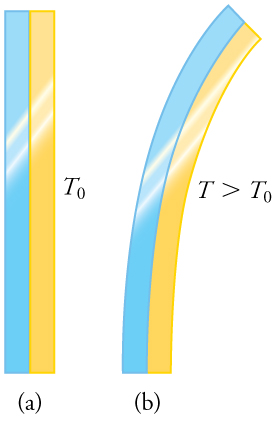Figure 1. The curvature of a bimetallic strip depends on temperature. (a) The strip is straight at the starting temperature, where its two components have the same length. (b) At a higher temperature, this strip bends to the right, because the metal on the left has expanded more than the metal on the right.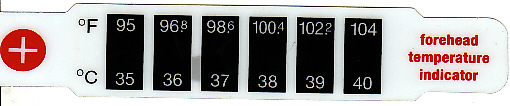Figure 2. Each of the six squares on this plastic (liquid crystal) thermometer contains a film of a different heat-sensitive liquid crystal material. Below 95ºF, all six squares are black. When the plastic thermometer is exposed to temperature that increases to 95ºF, the first liquid crystal square changes color. When the temperature increases above 96.8ºF the second liquid crystal square also changes color, and so forth. (credit: Arkrishna, Wikimedia Commons)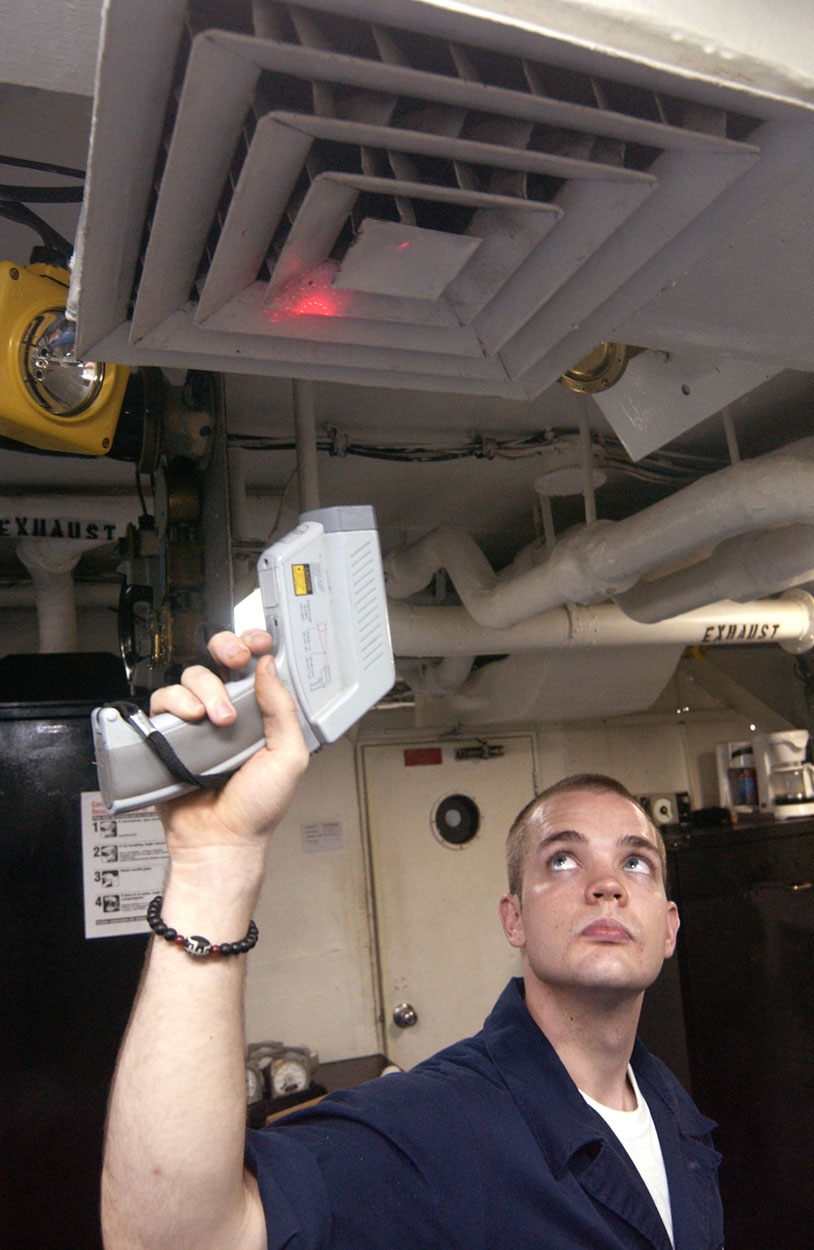Figure 3. Fireman Jason Ormand uses a pyrometer to check the temperature of an aircraft carrier’s ventilation system. Infrared radiation (whose emission varies with temperature) from the vent is measured and a temperature readout is quickly produced. Infrared measurements are also frequently used as a measure of body temperature. These modern thermometers, placed in the ear canal, are more accurate than alcohol thermometers placed under the tongue or in the armpit. (credit: Lamel J. Hinton/U.S. Navy)

# Temperature Scales

Thermometers are used to measure temperature according to well-defined scales of measurement, which use pre-defined reference points to help compare quantities. The three most common temperature scales are the Fahrenheit, Celsius, and Kelvin scales. A temperature scale can be created by identifying two easily reproducible temperatures. The freezing and boiling temperatures of water at standard atmospheric pressure are commonly used.

The Celsius scale (which replaced the slightly different centigrade scale) has the freezing point of water at$\boldsymbol{0^{\circ}\textbf{C}}$and the boiling point at$\boldsymbol{100^{\circ}\textbf{C}}.$Its unit is the degree Celsius$\boldsymbol{(^{\circ}\textbf{C})}.$On the Fahrenheit scale (still the most frequently used in the United States), the freezing point of water is at$\boldsymbol{32^{\circ}\textbf{F}}$and the boiling point is at$\boldsymbol{212^{\circ}\textbf{F}}.$The unit of temperature on this scale is the degree Fahrenheit$\boldsymbol{(^{\circ}\textbf{F}).}$Note that a temperature difference of one degree Celsius is greater than a temperature difference of one degree Fahrenheit. Only 100 Celsius degrees span the same range as 180 Fahrenheit degrees, thus one degree on the Celsius scale is 1.8 times larger than one degree on the Fahrenheit scale$\boldsymbol{180/100=9/5}.$

The Kelvin scale is the temperature scale that is commonly used in science. It is an absolute temperature scale defined to have 0 K at the lowest possible temperature, called absolute zero. The official temperature unit on this scale is the kelvin, which is abbreviated K, and is not accompanied by a degree sign. The freezing and boiling points of water are 273.15 K and 373.15 K, respectively. Thus, the magnitude of temperature differences is the same in units of kelvins and degrees Celsius. Unlike other temperature scales, the Kelvin scale is an absolute scale. It is used extensively in scientific work because a number of physical quantities, such as the volume of an ideal gas, are directly related to absolute temperature. The kelvin is the SI unit used in scientific work.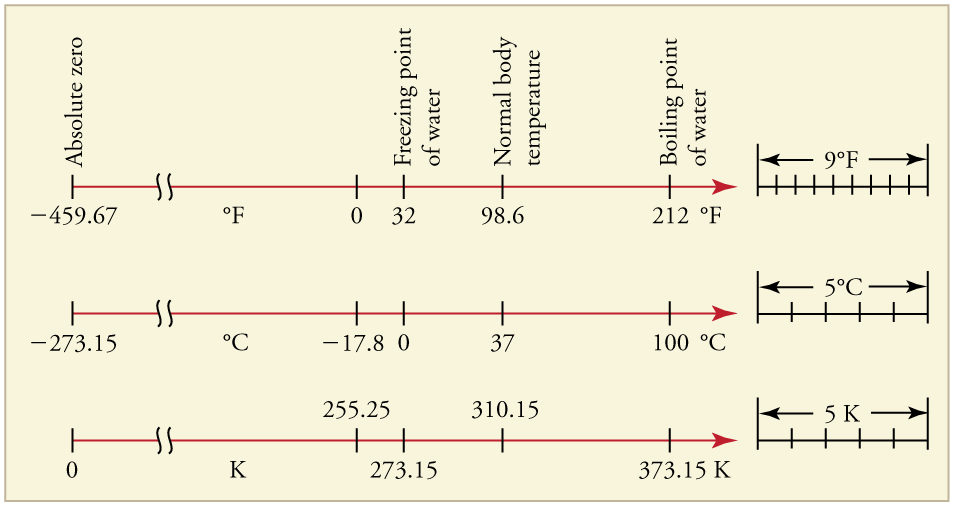Figure 4. Relationships between the Fahrenheit, Celsius, and Kelvin temperature scales, rounded to the nearest degree. The relative sizes of the scales are also shown.

The relationships between the three common temperature scales is shown in Figure 4. Temperatures on these scales can be converted using the equations in Table 1.

To convert from . . . Use this equation . . . Also written as . . .
Celsius to Fahrenheit $\boldsymbol{T(^{\circ}\textbf{F})=\frac{9}{5}T(^{\circ}\textbf{C})+32}$ $\boldsymbol{T_{^{\circ}\textbf{F}}=\frac{9}{5}T_{^{\circ}\textbf{C}}+32}$
Fahrenheit to Celsius $\boldsymbol{T(^{\circ}\textbf{C})=\frac{5}{9}T(^{\circ}\textbf{F})-32}$ $\boldsymbol{T_{^{\circ}\textbf{C}}=\frac{5}{9}(T_{^{\circ}\textbf{F}}-32)}$
Celsius to Kelvin $\boldsymbol{T(\textbf{K})=T(^{\circ}\textbf{C})+273.15}$ $\boldsymbol{T_{\textbf{K}}=T_{^{\circ}\textbf{C}}+273.15}$
Kelvin to Celsius $\boldsymbol{T(^{\circ}\textbf{C})=T(\textbf{K})-273.15}$ $\boldsymbol{T_{^{\circ}\textbf{C}}=T_{\textbf{K}}-273.15}$
Fahrenheit to Kelvin $\boldsymbol{T(\textbf{K})=\frac{5}{9}(T(^{\circ}\textbf{F})-32)+273.15}$ $\boldsymbol{T_{\textbf{K}}=\frac{5}{9}(T_{^{\circ}\textbf{F}}-32)+273.15}$
Kelvin to Fahrenheit $\boldsymbol{T(^{\circ}\textbf{F})=\frac{9}{5}T(\textbf{K})-273.15)+32}$ $\boldsymbol{T_{^\textbf{F}}=\frac{9}{5}(T_{\textbf{K}}-273.15)+32}$
Table 1. Temperature Conversions

Notice that the conversions between Fahrenheit and Kelvin look quite complicated. In fact, they are simple combinations of the conversions between Fahrenheit and Celsius, and the conversions between Celsius and Kelvin.

### Example 1: Converting between Temperature Scales: Room Temperature

“Room temperature” is generally defined to be$\boldsymbol{25^{\circ}\textbf{C}}.$(a) What is room temperature in$\boldsymbol{^{\circ}\textbf{F}}?$(b) What is it in K?

Strategy

To answer these questions, all we need to do is choose the correct conversion equations and plug in the known values.

Solution for (a)

1. Choose the right equation. To convert from$\boldsymbol{^{\circ}\textbf{C}}$to$\boldsymbol{^{\circ}\textbf{F}},$use the equation

$\boldsymbol{T_{^{\circ}\textbf{F}}\:=}$$\boldsymbol{\frac{9}{5}}$$\boldsymbol{T_{^{\circ}\textbf{C}}+32.}$

2. Plug the known value into the equation and solve:

$\boldsymbol{T_{^{\circ}\textbf{F}}\:=}$$\boldsymbol{\frac{9}{5}}$$\boldsymbol{25^{\circ}\textbf{C}+32=77^{\circ}\textbf{F}}.$

Solution for (b)

1. Choose the right equation. To convert from$\boldsymbol{^{\circ}\textbf{C}}$to K, use the equation

$\boldsymbol{T_{\textbf{K}}=T^{^{\circ}\textbf{C}}+273.15.}$

2. Plug the known value into the equation and solve:

$\boldsymbol{T_{\textbf{K}}=25^{\circ}\textbf{C}+273.15=298\textbf{K}}.$

### Example 2: Converting between Temperature Scales: the Reaumur Scale

The Reaumur scale is a temperature scale that was used widely in Europe in the 18th and 19th centuries. On the Reaumur temperature scale, the freezing point of water is$\boldsymbol{0^{\circ}\textbf{R}}$and the boiling temperature is$\boldsymbol{80^{\circ}\textbf{R}}.$If “room temperature” is$\boldsymbol{25^{\circ}\textbf{C}}$on the Celsius scale, what is it on the Reaumur scale?

Strategy

To answer this question, we must compare the Reaumur scale to the Celsius scale. The difference between the freezing point and boiling point of water on the Reaumur scale is$\boldsymbol{80^{\circ}\textbf{R}}.$On the Celsius scale it is$\boldsymbol{100^{\circ}\textbf{C}}.$Therefore$\boldsymbol{100^{\circ}\textbf{C}=80^{\circ}\textbf{R}}.$Both scales start at$\boldsymbol{0^{\circ}}$for freezing, so we can derive a simple formula to convert between temperatures on the two scales.

Solution

1. Derive a formula to convert from one scale to the other:

$\boldsymbol{T_{^{\circ}\textbf{R}}\:=}$$\boldsymbol{\frac{0.8^{\circ}\textbf{R}}{^{\circ}\textbf{C}}}$$\boldsymbol{\times\:T_{^{\circ}\textbf{C}}}.$

2. Plug the known value into the equation and solve:

$\boldsymbol{T_{^{\circ}\textbf{R}}\:=}$$\boldsymbol{\frac{0.8^{\circ}\textbf{R}}{^{\circ}\textbf{C}}}$$\boldsymbol{\times\:25^{\circ}\textbf{C}=20^{\circ}\textbf{R}}.$

# Temperature Ranges in the Universe

Figure 6 shows the wide range of temperatures found in the universe. Human beings have been known to survive with body temperatures within a small range, from$\boldsymbol{24^{\circ}\textbf{C}}$to$\boldsymbol{44^{\circ}\textbf{C}\:(75^{\circ}\textbf{F}}$to$\boldsymbol{111^{\circ}\textbf{F})}.$The average normal body temperature is usually given as$\boldsymbol{37.0^{\circ}\textbf{C}}$($\boldsymbol{98.6^{\circ}\textbf{F}}$), and variations in this temperature can indicate a medical condition: a fever, an infection, a tumor, or circulatory problems (see Figure 5).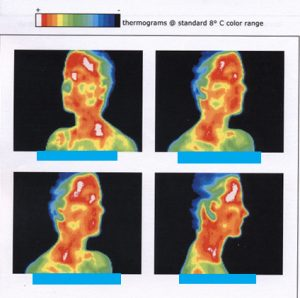Figure 5. This image of radiation from a person’s body (an infrared thermograph) shows the location of temperature abnormalities in the upper body. Dark blue corresponds to cold areas and red to white corresponds to hot areas. An elevated temperature might be an indication of malignant tissue (a cancerous tumor in the breast, for example), while a depressed temperature might be due to a decline in blood flow from a clot. In this case, the abnormalities are caused by a condition called hyperhidrosis. (credit: Porcelina81, Wikimedia Commons)

The lowest temperatures ever recorded have been measured during laboratory experiments:$\boldsymbol{4.5\times10^{-10}\textbf{ K}}$at the Massachusetts Institute of Technology (USA), and$\boldsymbol{1.0\times10^{-10}\textbf{ K}}$at Helsinki University of Technology (Finland). In comparison, the coldest recorded place on Earth’s surface is Vostok, Antarctica at 183 K$\boldsymbol{(-89^{\circ}\textbf{C})},$and the coldest place (outside the lab) known in the universe is the Boomerang Nebula, with a temperature of 1 K.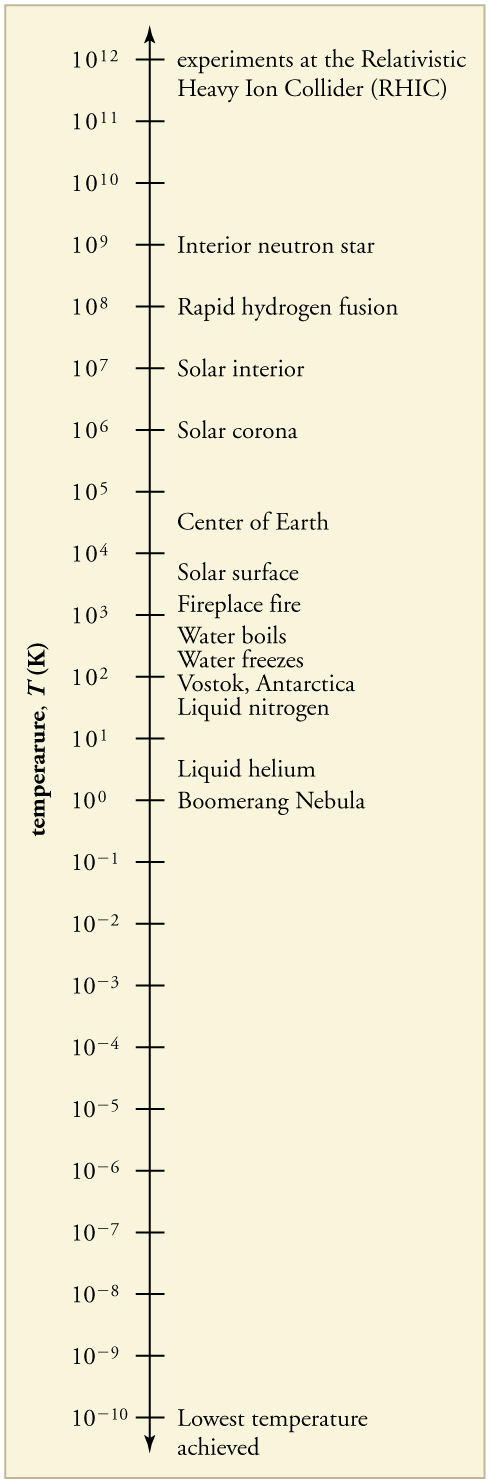Figure 6. Each increment on this logarithmic scale indicates an increase by a factor of ten, and thus illustrates the tremendous range of temperatures in nature. Note that zero on a logarithmic scale would occur off the bottom of the page at infinity.

### MAKING CONNECTIONS: ABSOLUTE ZERO

What is absolute zero? Absolute zero is the temperature at which all molecular motion has ceased. The concept of absolute zero arises from the behavior of gases. Figure 7 shows how the pressure of gases at a constant volume decreases as temperature decreases. Various scientists have noted that the pressures of gases extrapolate to zero at the same temperature,$\boldsymbol{-273.15^{\circ}\textbf{C}}.$This extrapolation implies that there is a lowest temperature. This temperature is called absolute zero. Today we know that most gases first liquefy and then freeze, and it is not actually possible to reach absolute zero. The numerical value of absolute zero temperature is$\boldsymbol{-273.15^{\circ}\textbf{C}}$or 0 K.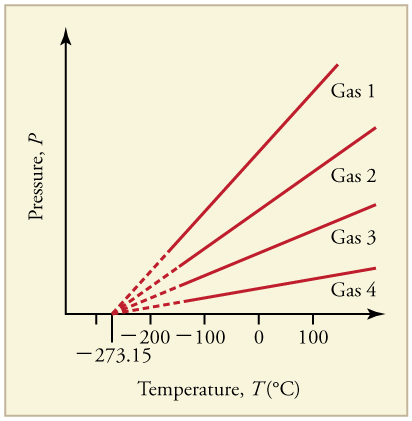Figure 7. Graph of pressure versus temperature for various gases kept at a constant volume. Note that all of the graphs extrapolate to zero pressure at the same temperature.

## Thermal Equilibrium and the Zeroth Law of Thermodynamics

Thermometers actually take their own temperature, not the temperature of the object they are measuring. This raises the question of how we can be certain that a thermometer measures the temperature of the object with which it is in contact. It is based on the fact that any two systems placed in thermal contact (meaning heat transfer can occur between them) will reach the same temperature. That is, heat will flow from the hotter object to the cooler one until they have exactly the same temperature. The objects are then in thermal equilibrium, and no further changes will occur. The systems interact and change because their temperatures differ, and the changes stop once their temperatures are the same. Thus, if enough time is allowed for this transfer of heat to run its course, the temperature a thermometer registers does represent the system with which it is in thermal equilibrium. Thermal equilibrium is established when two bodies are in contact with each other and can freely exchange energy.

Furthermore, experimentation has shown that if two systems, A and B, are in thermal equilibrium with each another, and B is in thermal equilibrium with a third system C, then A is also in thermal equilibrium with C. This conclusion may seem obvious, because all three have the same temperature, but it is basic to thermodynamics. It is called the zeroth law of thermodynamics.

### THE ZEROTH LAW OF THERMODYNAMICS

If two systems, A and B, are in thermal equilibrium with each other, and B is in thermal equilibrium with a third system, C, then A is also in thermal equilibrium with C.

This law was postulated in the 1930s, after the first and second laws of thermodynamics had been developed and named. It is called the zeroth law because it comes logically before the first and second laws (discussed in Chapter 15 Thermodynamics). An example of this law in action is seen in babies in incubators: babies in incubators normally have very few clothes on, so to an observer they look as if they may not be warm enough. However, the temperature of the air, the cot, and the baby is the same, because they are in thermal equilibrium, which is accomplished by maintaining air temperature to keep the baby comfortable.

### Check Your Understanding

1: Does the temperature of a body depend on its size?

## Section Summary

• Temperature is the quantity measured by a thermometer.
• Temperature is related to the average kinetic energy of atoms and molecules in a system.
• Absolute zero is the temperature at which there is no molecular motion.
• There are three main temperature scales: Celsius, Fahrenheit, and Kelvin.
• Temperatures on one scale can be converted to temperatures on another scale using the following equations:
$\boldsymbol{T_{^{\circ}\textbf{F}}\:=}$$\boldsymbol{\frac{9}{5}}$$\boldsymbol{T_{^{\circ}\textbf{C}}+32}$
$\boldsymbol{T_{^{\circ}\textbf{C}}\:=}$$\boldsymbol{\frac{5}{9}}$$\boldsymbol{(T_{^{\circ}\textbf{F}}-32)}$
$\boldsymbol{T_{\textbf{K}}=T_{^{\circ}\textbf{C}}+273.15}$
$\boldsymbol{T_{^{\circ}\textbf{C}}=T_{\textbf{K}}-273.15}$
• Systems are in thermal equilibrium when they have the same temperature.
• Thermal equilibrium occurs when two bodies are in contact with each other and can freely exchange energy.
• The zeroth law of thermodynamics states that when two systems, A and B, are in thermal equilibrium with each other, and B is in thermal equilibrium with a third system, C, then A is also in thermal equilibrium with C.

### Conceptual Questions

1: What does it mean to say that two systems are in thermal equilibrium?

2: Give an example of a physical property that varies with temperature and describe how it is used to measure temperature.

3: When a cold alcohol thermometer is placed in a hot liquid, the column of alcohol goes down slightly before going up. Explain why.

4: If you add boiling water to a cup at room temperature, what would you expect the final equilibrium temperature of the unit to be? You will need to include the surroundings as part of the system. Consider the zeroth law of thermodynamics.

### Problems & Exercises

1: What is the Fahrenheit temperature of a person with a$\boldsymbol{39.0^{\circ}\textbf{C}}$fever?

2: Frost damage to most plants occurs at temperatures of$\boldsymbol{28.0^{\circ}\textbf{F}}$or lower. What is this temperature on the Kelvin scale?

3: To conserve energy, room temperatures are kept at$\boldsymbol{68.0^{\circ}\textbf{F}}$in the winter and$\boldsymbol{78.0^{\circ}\textbf{F}}$in the summer. What are these temperatures on the Celsius scale?

4: A tungsten light bulb filament may operate at 2900 K. What is its Fahrenheit temperature? What is this on the Celsius scale?

5: The surface temperature of the Sun is about 5750 K. What is this temperature on the Fahrenheit scale?

6: One of the hottest temperatures ever recorded on the surface of Earth was$\boldsymbol{134^{\circ}\textbf{F}}$in Death Valley, CA. What is this temperature in Celsius degrees? What is this temperature in Kelvin?

7: (a) Suppose a cold front blows into your locale and drops the temperature by 40.0 Fahrenheit degrees. How many degrees Celsius does the temperature decrease when there is a$\boldsymbol{40.0^{\circ}\textbf{F}}$decrease in temperature? (b) Show that any change in temperature in Fahrenheit degrees is nine-fifths the change in Celsius degrees.

8: (a) At what temperature do the Fahrenheit and Celsius scales have the same numerical value? (b) At what temperature do the Fahrenheit and Kelvin scales have the same numerical value?

## Glossary

temperature
the quantity measured by a thermometer
Celsius scale
temperature scale in which the freezing point of water is$\boldsymbol{0^{\circ}\textbf{C}}$and the boiling point of water is$\boldsymbol{100^{\circ}\textbf{C}}$
degree Celsius
unit on the Celsius temperature scale
Fahrenheit scale
temperature scale in which the freezing point of water is$\boldsymbol{32^{\circ}\textbf{F}}$and the boiling point of water is$\boldsymbol{212^{\circ}\textbf{F}}$
degree Fahrenheit
unit on the Fahrenheit temperature scale
Kelvin scale
temperature scale in which 0 K is the lowest possible temperature, representing absolute zero
absolute zero
the lowest possible temperature; the temperature at which all molecular motion ceases
thermal equilibrium
the condition in which heat no longer flows between two objects that are in contact; the two objects have the same temperature
zeroth law of thermodynamics
law that states that if two objects are in thermal equilibrium, and a third object is in thermal equilibrium with one of those objects, it is also in thermal equilibrium with the other object

### Solutions

Check Your Understanding

1: No, the system can be divided into smaller parts each of which is at the same temperature. We say that the temperature is an intensive quantity. Intensive quantities are independent of size.

Problems & Exercises

1:

$\boldsymbol{102^{\circ}\textbf{F}}$

3:

$\boldsymbol{20.0^{\circ}\textbf{C}}$and$\boldsymbol{25.6^{\circ}\textbf{C}}$

5:

$\boldsymbol{9890^{\circ}\textbf{F}}$

7:

(a)$\boldsymbol{22.2^{\circ}\textbf{C}}$

(b)

$\begin{array}{lcl} \boldsymbol{\Delta{T}(^{\circ}\textbf{F})} & \boldsymbol{=} & \boldsymbol{T_2(^{\circ}\textbf{F})-T_1(^{\circ}\textbf{F})} \\ {} & \boldsymbol{=} & \boldsymbol{\frac{9}{5}T_2(^{\circ}\textbf{C})+32.0^{\circ}-(\frac{9}{5}T_1(^{\circ}\textbf{C})+32.0^{\circ})} \\ {} & \boldsymbol{=} & \boldsymbol{\frac{9}{5}T_2(^{\circ}\textbf{C})-T_1(^{\circ}\textbf{C})=\frac{9}{5}\Delta{T}(^{\circ}\textbf{C})} \end{array}$

## LicenseCollege Physics: OpenStax by OpenStax is licensed under a Creative Commons Attribution 4.0 International License, except where otherwise noted.